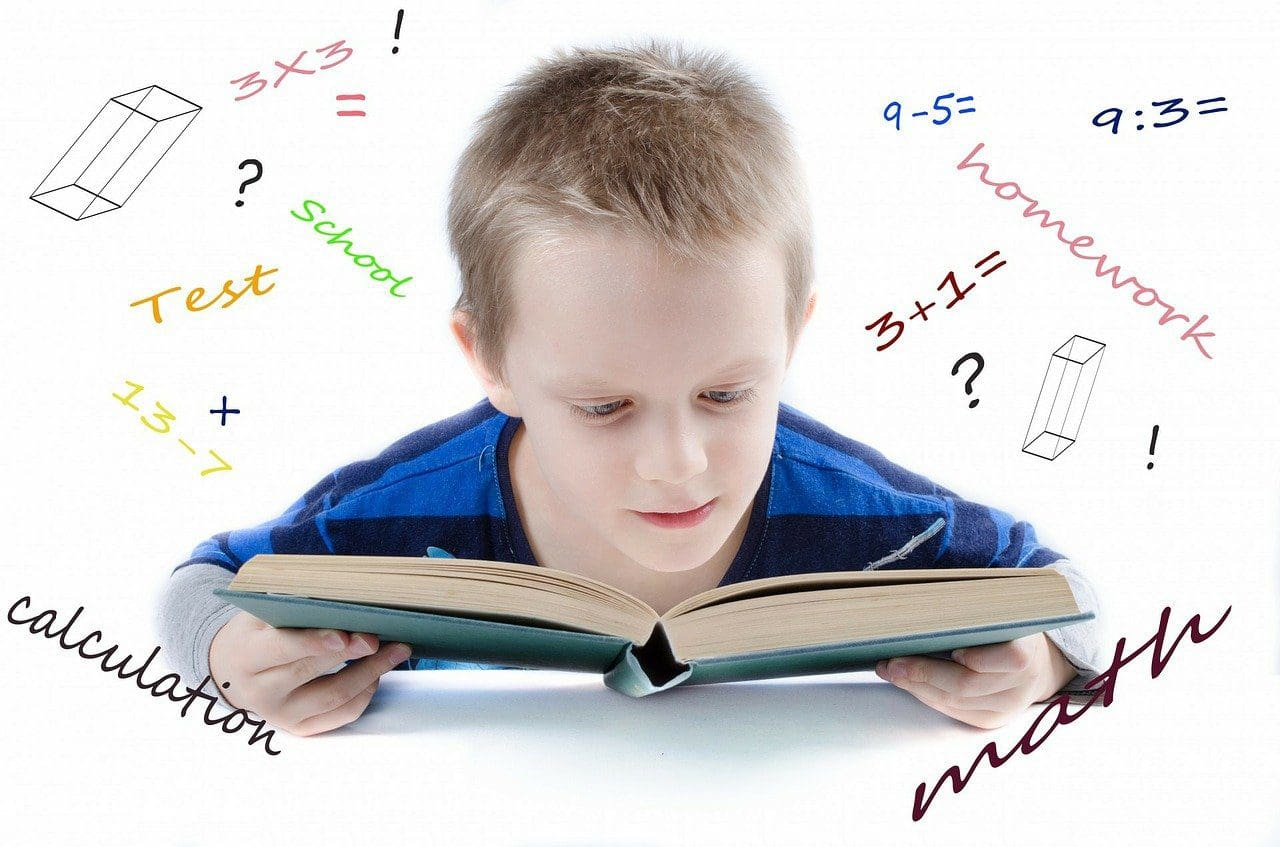Image by PublicDomainPicturs on Pixabay

## How are 4th Grade Math Worksheets Useful?

These math worksheets include problems on addition, subtraction, division, multiplication, roman numerals, decimals, telling time, etc. 4th grade math worksheets are very useful in developing concepts based on the above topics. Whenever a child is introduced to a new idea, he must make it a habit to solve worksheets related to that. It helps him to get a better understanding of that topic. In this article, we will look at how essential math worksheets are and the best worksheets available for kids in 4th grade.

## 1. Well-Organized

The problems available in the worksheets are very well structured. This means that the questions are arranged so that it helps kids build up their concepts gradually. These worksheets start with simple problems such as MCQs, match the following, and fill in the blanks. As kids solve these, they begin to understand how to apply the concept to questions. Once their confidence builds up, they can move on to solving more demanding sums that can include subjective sums, short and long answer questions, and so on. It helps kids greatly when they can attempt sums that are driven towards building a very strong mathematical foundation.